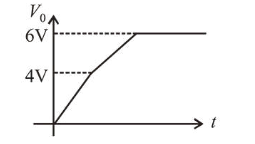# Two Zener diodes ( A and B ) having breakdown voltagesQuestion:

Two Zener diodes ( $A$ and $B$ ) having breakdown voltages of $6 \mathrm{~V}$ and $4 \mathrm{~V}$ respectively, are connected as shown in the circuit below. The output voltage $V_{0}$ variation with input voltage linearly increasing with time, is given by:

$\left(V_{\text {input }}=0 \mathrm{~V}\right.$ at $\left.t=0\right)$

(figures are qualitative)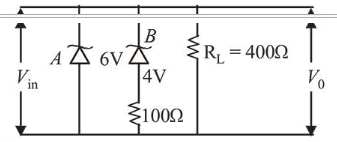1.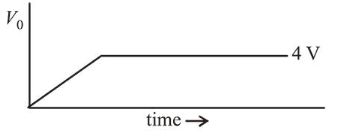2.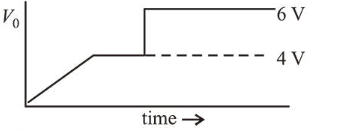3.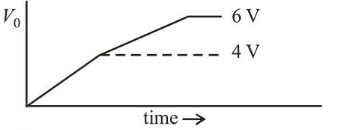4.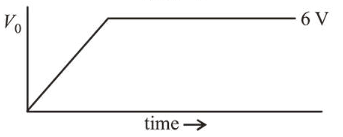Correct Option: , 3

Solution:

(3) Till input voltage reaches $4 \mathrm{~V}$. No zener is in breakdown region so $V_{0}=V_{i}$. Then now when $V_{i}$ changes between $4 \mathrm{~V}$ to $6 \mathrm{~V}$ one zener with $4 \mathrm{~V}$ will breakdown and P.D. across this zener will become constant and remaining potential will dorp across resistance in series with $4 \mathrm{~V}$ zener.

Now current in circuit increases abruptly and source must have an internal resistance due to which some potential will get drop across the source also so correct graph between $V_{0}$ and $t$ will be